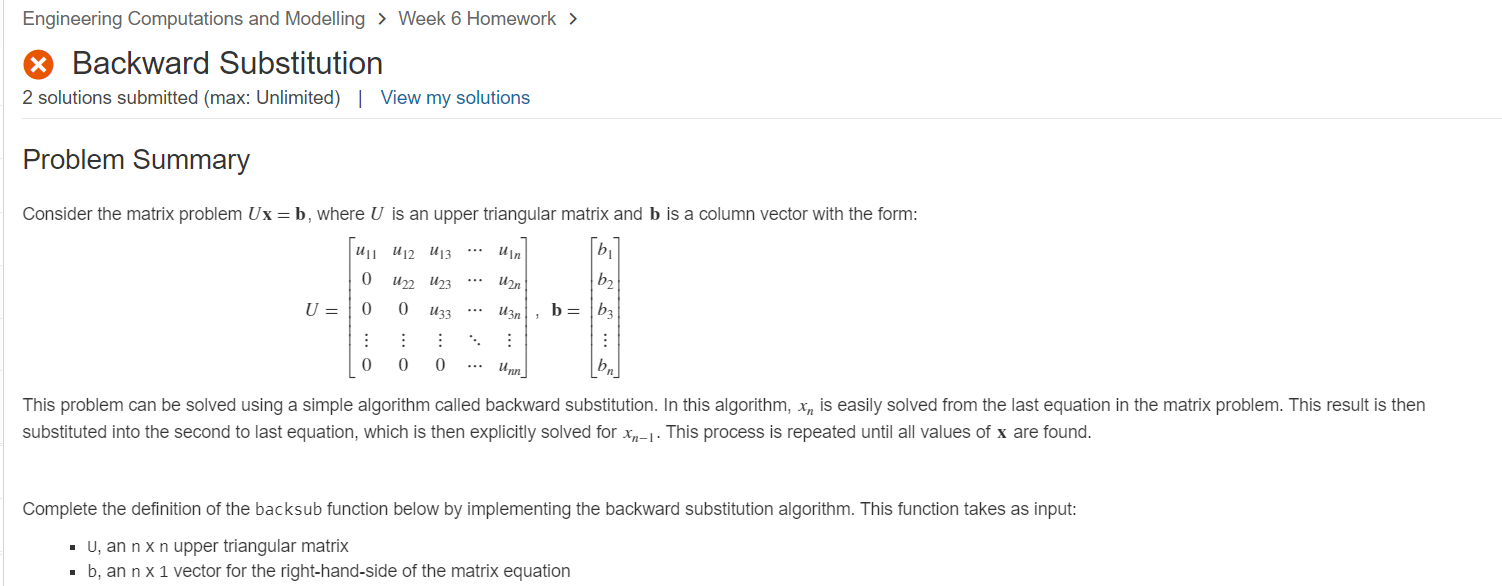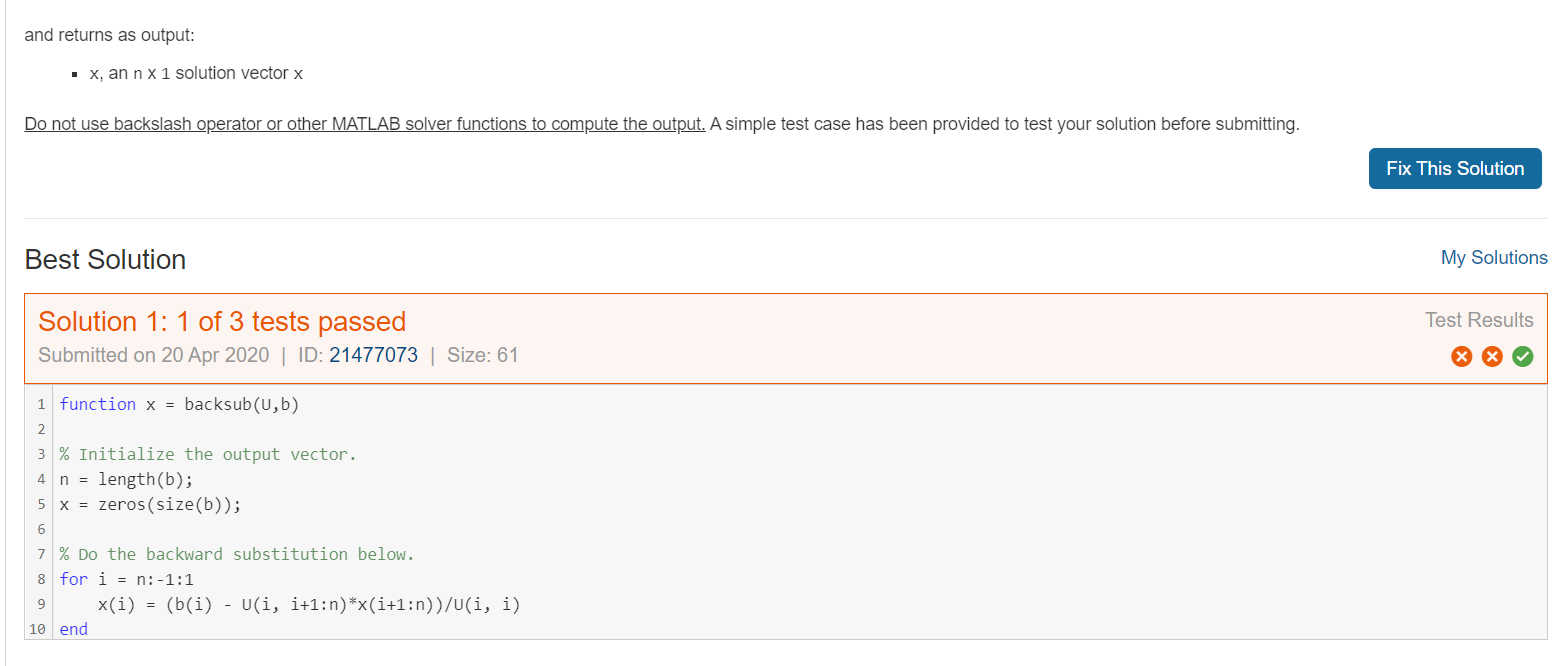# HERE IS THE CODE I FIXED BUT STILL DOESN'T WORK NOTE: THE VARIABLE x = zeros(size(b))...

###### Question:HERE IS THE CODE I FIXED BUT STILL DOESN'T WORK

NOTE: THE VARIABLE x = zeros(size(b)) CAN'T BE CHANGED CAUSE HAS BEEN SET BY ASSESSORHI EXPERTS I NEED HELP TO SOLVE THIS HOMEWORK PROBLEM FOR MATLAB CODE

A COUPLE OF TIMES I TRIED LAST TIME TO ASK BUT ALL OF THE ANSWERS WERE WRONG

PLEASE KINDLY HELP ME FIND THE RIGHT SOLUTION, ANY HELP WILL BE VERY APPRECIATE

Engineering Computations and Modelling > Week 6 Homework > Backward Substitution 2 solutions submitted (max: Unlimited) | View my solutions Problem Summary Consider the matrix problem Ux=b, where U is an upper triangular matrix and b is a column vector with the form: U11 U12 U13 U in bi 0 Uan b2 U22 U23 0 U33 U= 0 Uzn b= |b3 . ... ... ... ... 0 0 0 bn This problem can be solved using a simple algorithm called backward substitution. In this algorithm, xn is easily solved from the last equation in the matrix problem. This result is then substituted into the second to last equation, which is then explicitly solved for xn-1. This process is repeated until all values of x are found. Complete the definition of the backsub function below by implementing the backward substitution algorithm. This function takes as input: U, an nxn upper triangular matrix b, an nx 1 vector for the right-hand-side of the matrix equation
and returns as output: • X, an n x 1 solution vector x Do not use backslash operator or other MATLAB solver functions to compute the output. A simple test case has been provided to test your solution before submitting. Fix This Solution Best Solution My Solutions Test Results Solution 1: 1 of 3 tests passed Submitted on 20 Apr 2020 | ID: 21477073 | Size: 61 1 function x = backsub(0,b) 2 3 % Initialize the output vector. 4 n = length(b); 5 x = zeros(size(b)); 6 7 % Do the backward substitution below. 8 for i = n:-1:1 9 x(i) = (b(i) - U(i, i+1:n)*x(i+1:n))/(i, i) 10 end
Function C Reset LOMATLAB Documentation 1 function x = backsub(u,b) 2 3 % Initialize the output vector. 4 n = length(b); 5 x = zeros(size(b)); 6 7 % Do the backward substitution below. 8 for i = n:-1:1 9 S = 0 10 for j = 1:n 11 S = S + U(i, j)*x(j) 12 end 13 S = S - U(i, i)*x(i) 14 x(i) = (b(i) - s)/(i, i) 15 end 16 Code to call your function ® C Reset 1 U = [1 2;0 2]; 2 b = [2;1]; 3 X = backsub(0, b)

#### Similar Solved Questions

##### Find the function (fo h) and simplify. f(x) = 7x + 10, h(x) = Vx+6 (fo...
Find the function (fo h) and simplify. f(x) = 7x + 10, h(x) = Vx+6 (fo h)(x) = 12 (Simplify your answer.)...
##### 2 Tace the path of a drop of venous blood from the left ankle to the...
2 Tace the path of a drop of venous blood from the left ankle to the right side of the brain...